# 傅里叶变换终极必杀技

1. 采样定理（采样频率至少要大于原始信号最高频率的2倍才能提取出原始信号信息）；
2. 欧拉公式的应用: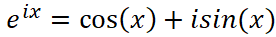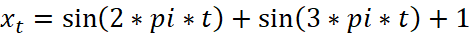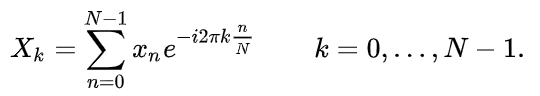第一个点：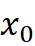= sin(2*pi*0) + sin(3*pi*0) + 1 = 1

第二个点：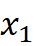= sin(2*pi*0.25) + sin(3*pi*0.25) + 1 =   2.7071

第三个点：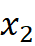= sin(2*pi*0.5) + sin(3*pi*0.5) + 1 =   0

第四个点：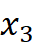= sin(2*pi*0.75) + sin(3*pi*0.75) + 1 =   0.70711

第五个点：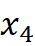= sin(2*pi*1) + sin(3*pi*1) + 1 =   1

第六个点：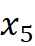= sin(2*pi*1.25) + sin(3*pi*1.25) + 1 =   1.2929

第七个点：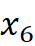= sin(2*pi*1.5) + sin(3*pi*1.5) + 1 =   2

第八个点：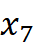= sin(2*pi*1.75) + sin(3*pi*1.75) + 1 =   -0.70711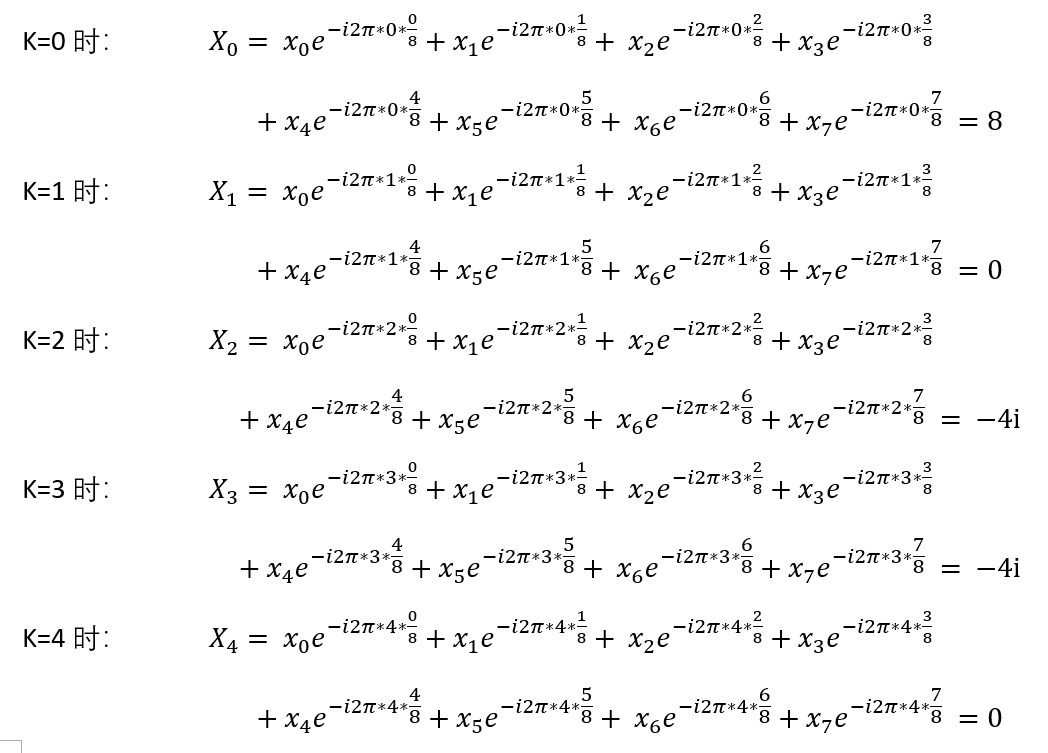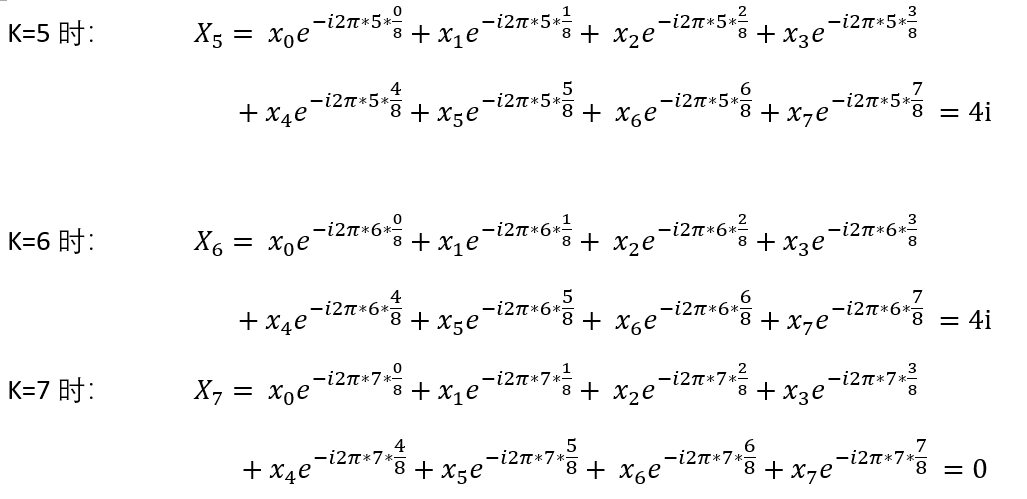（虽然看起来上面的计算狠复杂（其实真的很复杂呀），但至少上面没有未知数，结果是一定存在的，不管是按计算器，matlab工具，或者欧拉公式，只有能计算出正确结果，就是好方法。）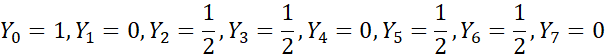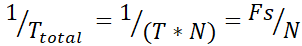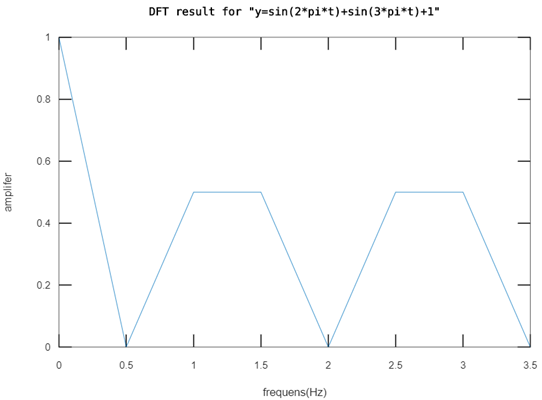（对称的理论证明，传送门在此：https://www.zhihu.com/question/264560305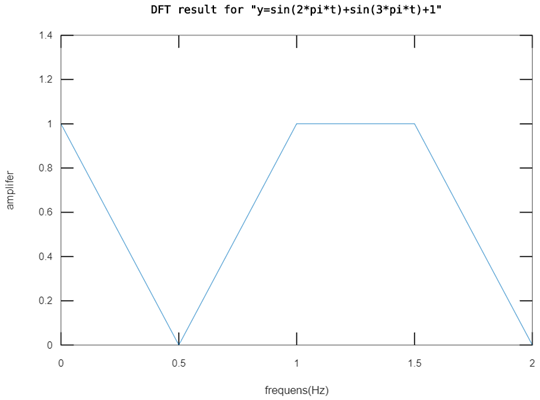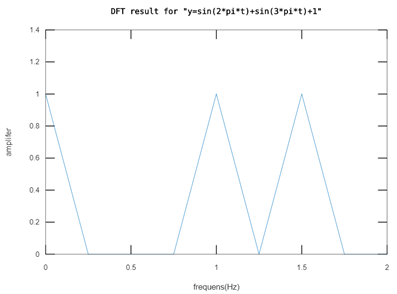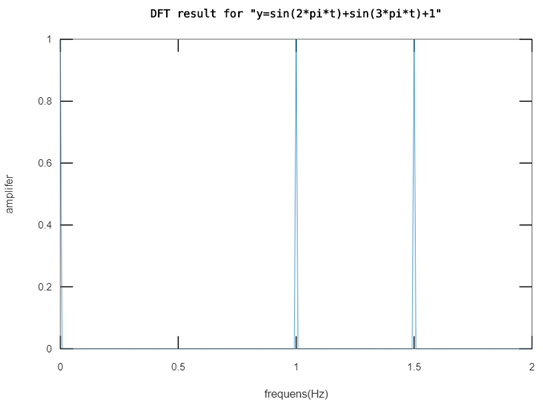-------------------------程序开始（可在线运行https://octave-online.net/ ）------------------------------

Fs = 4;                                % 设置采样率

T = 1/Fs;                            % 采样周期

N = 512;                            % 设置采样点数，设为8即可得到上面的实验结果

t = (0:N-1)*T;                   % 确定采样值，: 表示变量的取值范围

%t                                       % matlab中变量不加分号时会打印出变量的值

y = sin(2*pi*t)+sin(3*pi*t)+1;     % 本例子的原始信号，可以看出f = 0Hz, f=1Hz, f=1.5Hz 的信号分量

%y

Y = fft(y);                           % FFT是通过矩阵计算傅里叶变换的一种算法，和DFT结果是一样的

%Y                                      % 把Y前面的百分号去掉，就可以快速得到上面的计算结果啦！

Y1 = Y/N;                           % 上面有说，我也不知道为啥要除以N

%Y1

P2 = abs(Y1);                    % 求复数的模长

%P2

%f = Fs*(0:N-1)/N;          % 频率分辨率为Fs/N,  频率范围是0到Fs(N-1)/N

%plot(f,P2);                      % 以f为x轴坐标的格点，画出对称的变换结果

%xlabel('frequens(Hz)');              % 设置x轴坐标名称

%ylabel('amplifer');                      % 设置y轴坐标名称

%title('DFT result for \"y=sin(2*pi*t)+sin(3*pi*t)+1\"');                 % 设置图像标题

P1 = P2(1:N/2+1);                          % 把对称的部分图形去掉

%P1

P1(2:end-1) = 2*P1(2:end-1);      % 从第二个结果开始幅值都加倍（end表示最后一个值）

%P1

f = Fs*(0:N/2)/N;                           % 频率分辨率为Fs/N,  频率范围是0到Fs/2

%f

plot(f,P1);                                       %以f为x轴坐标的格点，画出去掉对称部分的变换结果

xlabel('frequens(Hz)');

ylabel('amplifer');

title('DFT result for \"y=sin(2*pi*t)+sin(3*pi*t)+1\"');

-------------------------------------------------程序完------------------------------------------------------------

https://ww2.mathworks.cn/help/matlab/ref/fft.html

©️2019 CSDN 皮肤主题: 大白 设计师: CSDN官方博客